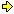World!Of
NumbersWON plate
160 |

[ October 18, 2004 ]
Two Prime Numbers starting from 18 and 19
Farideh Firoozbakth (email)

Farideh Firoozbakht from Iran
found two nice probable primes  p1 and p2
formed by concatenating the integers
from 18 resp. 19 up to 3607 resp. 1471.

181920... ...36063607 (13296 digits)
and
192021... ...14701471 (4750 digits)

hitherto unknown in the prime number community.
Note that the endnumbers 1471 and 3607 of the strings
are primes, as well as 'their' concatenation 14713607 !

Farideh found these primes by usingMathematicathat she
masters during 15 years now. "It is as necessary to me as air"
she writes in one of her messages.

Mathematica says p1 & p2 are primes, i.e.,

In:= {PrimeQ[p1],PrimeQ[p2]}

Out= {True, True}

Note that the built-in primality testing function PrimeQ
does not actually give a proof that a number is prime.
However, there are no known examples where PrimeQ fails.

In Mathematica we can generate p1 & p2 in this way :

p1=(v1={};Do[v1=Join[v1,IntegerDigits[k]],{k,18,3607}];FromDigits[v1])

p2=(v1={};Do[v1=Join[v1,IntegerDigits[k]],{k,19,1471}];FromDigits[v1])

And Mathematica determines length of p1 & p2 in this way:

In:= Length[IntegerDigits[p1]]
Out= 13296

In:= Length[IntegerDigits[p2]]
Out= 4750

Of course there is a limit with Mathematica but I think
the limit also depends on the computer that we use.
Mathematica can work on numbers with more than 30000000 digits.
For example:

In:=
n=100000000;Timing[{Length[IntegerDigits[2^n]],DigitCount[2^n]}]

Out=
{1185.36 Second,{30103000,{3009550,3011051,3005571,
3011482,3011589,3010173,3010805,3011875,3010874,3010030}}}

This means that Mathematica counts the digits of m = 2^100000000
and counts the digits 0 to 9 of m in 1185.36 seconds.
Indeed Mathematica says m is a 30103000-digit number and :

k — number of k in m

0 — 3009550
1 — 3011051
2 — 3005571
3 — 3011482
4 — 3011589
5 — 3010173
6 — 3010805
7 — 3011875
8 — 3010874
9 — 3010030With the aid of the freeware tool PFGW (PrimeForm)
I (PDG) could verify Farideh's results.
PFGW has a built-in function for Smarandache sequences 'Sm'.
Using the double coordinates Sm(x,y) makes for a circular
shift starting from y, so I had to divide the string with
the length of the shifted part. PFGW always rounds up
till an integer is left over. Reals cannot be input evidently.
Here are the commands and timings on my system
(Pentium 4 at 3 GHz) :C:\PFGW>pfgw -q"sm(1471,19)/10^len(sm(18))" PFGW Version 20040816.Win_Stable (v1.2 RC1a) [FFT v23.8] sm(1471,19)/10^len(sm(18)) is 3-PRP! (1.5122s+0.0020s)

 C:\PFGW>pfgw -q"sm(3607,18)/10^len(sm(17))" PFGW Version 20040816.Win_Stable (v1.2 RC1a) [FFT v23.8] sm(3607,18)/10^len(sm(17)) is 3-PRP! (15.6343s+0.0051s)

The lengths of the numbers can be determined as follows :

 C:\PFGW>pfgw -od -f0 -q"len(sm(1471,19)/10^27)" PFGW Version 20040816.Win_Stable (v1.2 RC1a) [FFT v23.8] No factoring at all, not even trivial division len(sm(1471,19)/10^27): 4750

 C:\PFGW>pfgw -od -f0 -q"len(sm(3607,18)/10^25)" PFGW Version 20040816.Win_Stable (v1.2 RC1a) [FFT v23.8] No factoring at all, not even trivial division len(sm(3607,18)/10^25): 13296The Reference Table for
Smarandache Concatenated Numbers
with starting numbers from 1 to 19.
Maybe you like to participate ?

 Startfrom Smarandache Concatenated NumbersList of Probable Primesdigitlength Searched up tolast term 1 nihil 1504164099 2 32, 98, 2744 10047 3 1927 10067 4 74, 1314 10259 5 1721, 71129, 99185, 123257 10351 6 72, 1312 10355 7 1311, 127267, 9492733, 717127571 PDG 10001[ 7171 dd. 25/10/2004 ] 8 92, 149332 10135 9 187445 10265 10 nihil 12701 11 309808 11041 12 134 10091 13 729728066 PDG 10265[ 7297 dd. 23/10/2004 ] 14 178, 4768 10089 15 1910 10469 16 4356 15677 17 3946 10077 18 360713296 FF 11375 19 14714750 FF 10901Entries of F. Firoozbakht in Prime Curios!
Primes by Listing
Consecutive Number SequencesA000160Prime Curios!Prime PuzzleWikipedia 160Le nombre 160```

```

[TOP OF PAGE]

Patrick De Geest - Belgium- Short Bio - Some Pictures